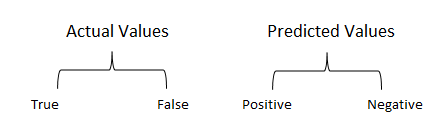# Simple Guide to the confusion matrix

A confusion matrix is a table that is often used to describe the performance of the classification model (or “classifier”) on a set of test data for which the true values are known. The confusion matrix itself is relatively simple to understand, but the related terminology can be confusing.

Confusion matrix

A classification problem can be evaluated by comparing the predicted labels to the actual labels using a confusion matrix.Each prediction can be one of the four outcomes, based on how it matches up to the actual value:

• True Positive (TP): Correctly predicted to be a positive class.
• False Positive (FP): Incorrectly predicted to be a positive class.
• True Negative (TN): Correctly predicted to not be a positive class.
• False Negative (FN): Incorrectly predicted to not be a positive class.

False positives are also referred to as type I errors, while false negatives are type II errors. Given a certain classifier, an effort to reduce one will cause an increase in the other.

Let’s understand TP, FP, FN, TN in terms of pregnancy analogy.Just remember, we describe predicted values as Positive and Negative and actual values as True and False.The below is a list of rates that are often computed from a confusion matrix for a classifier:

Accuracy

When our classes are roughly equal in size, we can use accuracy, which will give us correctly classified values:Error rate or Misclassification rate

Since accuracy is the percent we correctly classified (success rate), it follows that our error rate (the percentage we got wrong) can be calculated as follows:Precision

When we have a class imbalance, accuracy can become an unreliable metric for measuring our performance. For instance, if we had a 99/1 split between two classes, A and B, where the rare event, B, is our positive class, we could build a model that was 99% accurate by just saying everything belonged to class A. Clearly, we shouldn’t bother building a model if it doesn’t do anything to identify class B; thus, we need different metrics that will discourage this behaviour. For this, we use precision and recall instead of accuracy. Precision tells us about the ratio of true positives to everything flagged positively:Recall

Recall gives us the true positive rate (TPR), which is the ratio of true positives to everything that was actually positive:In the case of the 99/1 split between classes A and B, the model that classifies everything as A would have a recall of 0% for the positive class, B (precision would be undefined—0/0). Precision and recall provide a better way of evaluating model performance in the face of a class imbalance. They will correctly tell us that the model has little value for our use case.

Just like accuracy, both precision and recall are easy to compute and understand but require thresholds. In addition, precision and recall only consider half of the confusion matrix:F Score

The classification report also includes the F1 score, which helps us balance precision and recall using the harmonic mean of the two:Sensitivity and Specificity

Sensitivity is the true positive rate, or recall, which we saw previously. Specificity, however, is the true negative rate, or the proportion of true negatives to everything that should have been classified as negative:Note that, together, specificity and sensitivity consider the full confusion matrix:ROC Curve

In addition to using metrics to evaluate classification problems, we can turn to visualizations. By plotting the true positive rate (sensitivity) versus the false-positive rate (1 - specificity), we get the Receiver Operating Characteristic (ROC) curve. This curve allows us to visualize the trade-off between the true positive rate and the false positive rate.

The following are examples of good ROC curves. The dashed line would be random guessing (no predictive value) and is used as a baseline; anything below that is considered worse than guessing. We want to be toward the top-left corner:Here is a python code which shows how to make a confusion matrix on an anticipated model. For this, we need to import the confusion matrix module from the sklearn library which encourages us to create the confusion matrix.Output:I hope I’ve given some understanding of the confusion matrix and terminology used. If you like this post, please do share on Facebook, Twitter, Linkedin and don’t forget to subscribe to get the latest updates from the blog.